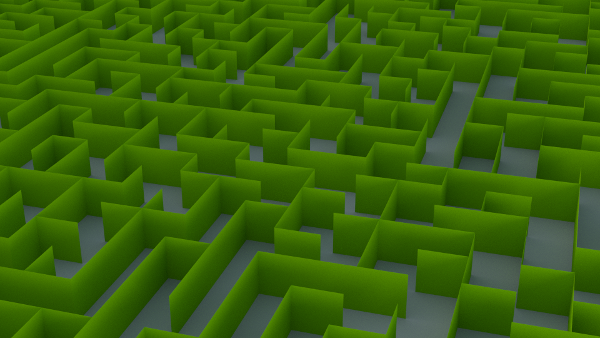## blender labyrinth generator

I ported my labyrinth generator from processing to a blender python scriptfrom random import shuffle from array import * import bpy

``````N=1
S=2
E=4
W=8

odir = {}
odir[N] = S
odir[S] = N
odir[W] = E
odir[E] = W

WIDTH=40
HEIGHT=40

coords = []
faces = []

d = [[0 for col in range(WIDTH)] for row in range(HEIGHT)]

for i in range(0,WIDTH):
for j in range(0, HEIGHT):
d[i][j] = 0

def carve( x, y ):
dirs = [N,S,W,E]
shuffle(dirs)

for i in dirs:
print(i)
nx = x
if i == E:
nx =  nx + 1
if i == W:
nx = nx - 1
ny = y
if i == S:
ny = ny +1
if i == N:
ny = ny - 1
if nx >= 0 and nx < WIDTH and ny >=0 and ny < HEIGHT and d[nx][ny] == 0:
d[x][y] = d[x][y] | i
d[nx][ny] = d[nx][ny]  | odir[i]
carve(nx, ny)

carve(0,0)

idx=0
step = 0.1

for x in range(0,WIDTH):
for y in range( 0, HEIGHT ):
if ( d[x][y] & N ) == 0:
coords.append((x * step , y * step, 0 ))
coords.append(((x+1) * step, y * step, 0 ))
coords.append(((x+1) * step, y * step, step ))
coords.append((x * step, y * step, step ))
faces.append(( idx, idx+1, idx+2, idx+3))
idx+=4
if ( d[x][y] & S ) == 0:
coords.append((x * step , (y+1) * step, 0 ))
coords.append(((x+1) * step, (y+1) * step, 0 ))
coords.append(((x+1) * step, (y+1) * step, step ))
coords.append((x * step, (y+1) * step, step ))
faces.append(( idx, idx+1, idx+2, idx+3))
idx+=4
if ( d[x][y] & E ) == 0:
coords.append(((x+1) * step, y * step, 0 ))
coords.append(((x+1) * step, (y+1) * step, 0 ))
coords.append(((x+1) * step, (y+1) * step, step ))
coords.append(((x+1) * step, y * step, step ))
faces.append(( idx, idx+1, idx+2, idx+3))
idx+=4
if ( d[x][y] & W ) == 0:
coords.append((x * step, y * step, 0 ))
coords.append((x * step, (y+1) * step, 0 ))
coords.append((x * step, (y+1) * step, step ))
coords.append((x * step, y * step, step ))
faces.append(( idx, idx+1, idx+2, idx+3))
idx+=4

mesh = bpy.data.meshes.new(name="MyMesh")

object = bpy.data.objects.new( 'MESH', mesh )

mesh.from_pydata( coords, [], faces )
mesh.update( calc_edges=True )
``````

Leave a response

1. The program crash when you call carve(0,0)

2. Is it possible to adjust the width of the paths? Because I'm trying to make an object pathfind through it.

3. Hello, Can it be used for commercially? To produce games ...

4. @Júnior - yes. But I would love it if you give credits and add a link to it somewhere (for example in an about screen, download site, etc. )

5. Hi, could you please explain the code? I really can't get some fragments.. for Ex. odir = {} odir[N] = S odir[S] = N odir[W] = E odir[E] = W

What does this do? Thank you in advance.

6. The odir map stores the opposite directions - for example the opposite direction for "North" is "South" so odir[N] = S and so on